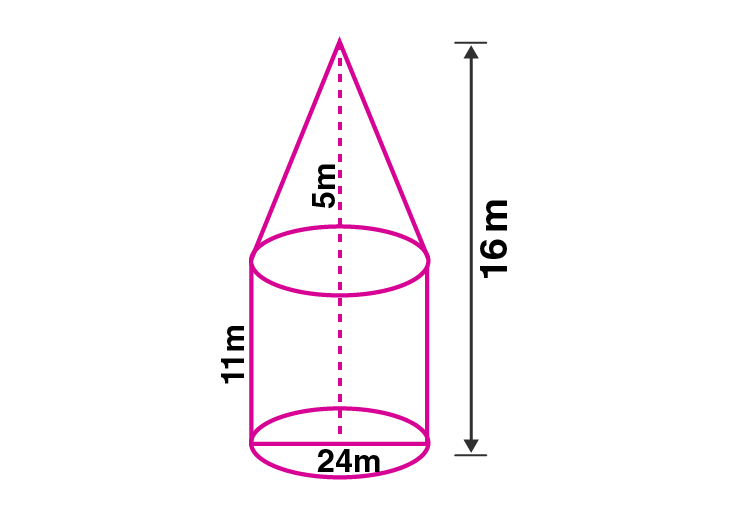Newbie

# A circus tent is in the shape of a cylinder surmounted by a cone. The diameter of the cylindrical portion is 24 m and its height is 11 m. If the vertex of the cone is 16 m above the ground, find the area of the canvas used to make the tent.

• 1

M.L Aggarwal book Important Question of class 10 chapter Based on Mensuration for ICSE BOARD.
A circus tent is in the shape of a cylinder surmounted by a cone. The diameter of the cylindrical portion and its height is given. If the vertex of the cone is 16 m above the ground, find the area of the canvas used to make the tent.
This is the Question Number 10, Exercise 17.4 of M.L Aggarwal.

Share

1.Given diameter of the cylindrical part of tent, d = 24 m

Radius, r = d/2 = 24/2 = 12 m

Height of the cylindrical part, H = 11 m

Since vertex of cone is 16 m above the ground, height of cone, h = 16-11

h = 5 m

Slant height of the cone, l = √(h2+r2)

= √(52+122)

= √(25+144)

= √(169)

= 13 m

Radius of cone, r = 12 m

Area of canvas used to make the tent = curved surface area of the cylindrical part + curved surface area of the cone.

Area of canvas used to make the tent = 2rH+rl

= r(2H+l)

= (22/7)×12×(2×11+13)

= (264/7)×(22+13)

= (264/7)×35

= 264×5

= 1320 m2

Hence the area of canvas used to make the tent is 1320 m2 .

• 1